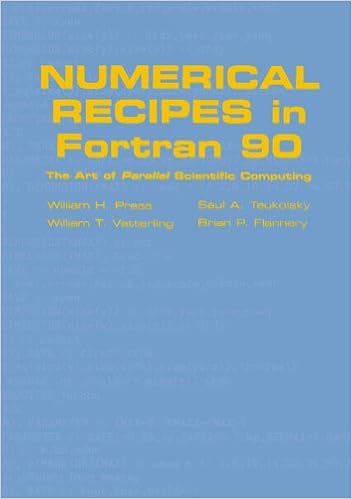# Fortran Wiki Numerical Recipes in Fortran 90 - The Art of Parallel Scientific ComputingBy William H. Press, Saul A. Teukolsky, William T. Vetterling, Brian P. Flannery, Michael Metcalf (1996)
Cambridge University Press## Description

Numerical Recipes in Fortran 90 starts with a detailed introduction to the Fortran 90 language and then presents the basic concepts of parallel programming. All 350+ routines from the second edition of Numerical Recipes are presented in Fortran 90. Many are completely reworked algorithmically so as to be ‘parallel-ready’ and to utilise Fortran 90’s advanced language features. Numerical Recipes in Fortran 90 emphasises general principles, but throughout there are also numerous hints and tips. This volume is intended for use with the original Numerical Recipes in Fortran, 2nd Edition (now called Numerical Recipes in Fortran 77) and does not discuss how the individual programs are used, or how the mathematical methods are used. An excellent guide for Fortran programmers interested in moving to Fortran 90, or C/C++ programmers interested in parallel programming.

## Contents

• Introduction to Fortran 90 language features
• Introduction to parallel programming
• Numerical recipes utility functions for Fortran 90

Part I. Fortran 90 Code chapters:

• Preliminaries
• Solution of linear algebraic equations
• Interpolation and extrapolation
• Integration of functions
• Evaluation of functions
• Special functions
• Random numbers
• Sorting
• Root finding and nonlinear sets of equations
• Minimization or maximization of functions
• Eigensystems
• Fast Fourier transform
• Fourier and spectral applications
• Statistical description of Data
• Modelling of data
• Integration of ordinary differential equations
• Two point boundary value problems
• Integral equations and inverse theory
• Partial differential equations
• Less-numerical algorithms

Part II. Appendices:

• Listing of utility modules (nrtype and nrutil)
• Listing of explicit interfaces
• Index of programs and dependencies

category: books